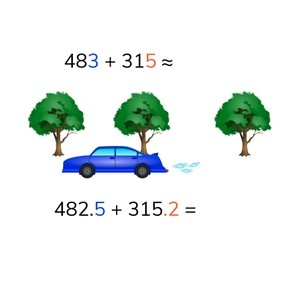Estimated and exact sum with decimal numbers with 1 or 2 decimal places

# Estimated and exact sum with decimal numbers with 1 or 2 decimal places

No account needed.8,000 schools use Gynzy92,000 teachers use Gynzy1,600,000 students use Gynzy

## General

Students learn to estimate the total and find the exact total of decimal numbers with 1 or 2 decimal places.

5.NBT.B.7

## Relevance

It is useful to be able to add decimal numbers with 1 or 2 decimal places exactly, and to be able to estimate the sum, so you can use the estimation as a check for the exact total. You need this if you want to know, for example, about how much you have to pay.

## Introduction

The interactive whiteboard shows a number line from 0 to 1 and a few decimal numbers are given. The students must determine where the numbers belong on the number line and draw a line to that location. Emphasize that numbers with two decimal places is not always bigger than a number with 1 decimal place.

## Development

To check that students are able to add decimal numbers with 1 or 2 decimals and can give an estimated and exact sum, you can ask the following questions:
- How do you round the decimal numbers to estimate the sum?
- How do you add decimal numbers if you want to know the exact total?
- How do you know if the exact answer is correct?

## Guided practice

Students first practice adding decimal numbers with 1 decimal place. Then they practice adding decimal numbers with 2 decimal places. They are finally asked to solve both kinds in story form.

## Closing

Discuss with students that it is important to be able to estimate the sum so you can check your exact sum as well- as these totals should be close to each other. Check that students are able to estimate the sum and determine the exact sum by splitting the second addend, or by splitting both addends by asking them how they would solve the given addition problems. They are then given two receipts with two purchased items. Students must calculate the estimated and exact sums.
--!
!---Teaching tips
If students have difficulty with this goal, they can use the number line to add the decimal numbers together, potentially using smaller jumps to add the numbers together.

### The online teaching platform for interactive whiteboards and displays in schools

• Save time building lessons

• Manage the classroom more efficiently

• Increase student engagement